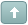Error

# Re: Saving as SVG generates a very small sketch

#1 Posted : Tuesday, August 30, 2005 12:23:11 PM(UTC)

I am converting a bunch of polylines into an SVG file, and at the beginning of the file I am setting the L_VecSetParallelogram (@Vh, @Min, @Max) to the maximum extents of the sketch.

However when I load this file in Adobe's SVG viewer, or even Amaya, the lines are drawn very small and in the upper corner, as if the scale is way off.  What is the proper way for exploring this?

Thanks!

 Try the latest version of LEADTOOLS for free for 60 days by downloading the evaluation: https://www.leadtools.com/downloadsWanna join the discussion? Login to your LEADTOOLS Support accountor Register a new forum account.#2 Posted : Monday, September 5, 2005 12:28:46 AM(UTC)
Hello,

What is the LEADTOOLS version that you are using?
Can you provide a small code snippet that shows how exactly you are saving you’re polylines to SVG file?

Thanks,

#3 Posted : Wednesday, September 7, 2005 9:24:31 AM(UTC)
I am using LeadTools v14.  My code snippet is as follows, please let me know if you don't understand a part of the conversion.

TG_POLYLINE : begin
FillChar (Vo, SizeOf(Vo), #0);
L_VecInitObject (@Vo.VecObject);

if PTG_POLYLINE_ENT(E^.data)^.vertices^.count = 2 then begin
// free form line
Vo.VecObject.nSize := SizeOf(VECTORPOLYLINE);
Vo.VecObject.nType := VECTOR_POLYLINE;

Vo.nPointCount := PTG_POLYLINE_ENT(E^.data)^.vertices^.count;
if Vo.nPointCount > 0 then begin

GetMem (Vo.Point, SizeOf(VECTORPOINT)*Vo.nPointCount);
x := 1;

with PTG_POLYLINE_ENT(E^.data)^ do begin

repeat

PVECTORPOINTARRAY(Vo.Point)^[x].x := PTG_VERTEX_ENT(PTG_ENTITY(vertices^.current^.data)^.data)^.pnt.x;

PVECTORPOINTARRAY(Vo.Point)^[x].y := PTG_VERTEX_ENT(PTG_ENTITY(vertices^.current^.data)^.data)^.pnt.y;

PVECTORPOINTARRAY(Vo.Point)^[x].z := PTG_VERTEX_ENT(PTG_ENTITY(vertices^.current^.data)^.data)^.pnt.z;

Inc (x);

until ll_set_list_currpntr (vertices, TG_AFTER) = TG_HEAD;
end; { with }
end; { if }

T.Pen.nSize := SizeOf(VECTORPEN);
T.Pen.bExtPen := TRUE;
T.Pen.Pen.elpWidth := 1;
T.Pen.Pen.elpBrushStyle := BS_HOLLOW;
T.Pen.Pen.elpColor := ColorToRGBColor(E^.Color);
T.Pen.Pen.elpPenStyle := PS_SOLID;
T.Brush.nSize := SizeOf(VECTORBRUSH);
T.Brush.VectorBrushStyle := VECTORBRUSH_STANDARD;

T.Brush.StandardBrush.LogicalBrush.lbStyle := BS_HOLLOW;

{ShowMessage (IntToStr (}L_VecAddObject (@Vh, @Lyr, VECTOR_POLYLINE, @Vo, nil){))};
end { if... }
else begin
// area
Vo.VecObject.nSize := SizeOf(VECTORPOLYGON);
Vo.VecObject.nType := VECTOR_POLYGON;

Vo.nPointCount := PTG_POLYLINE_ENT(E^.data)^.vertices^.count;
if Vo.nPointCount > 0 then begin

GetMem (Vo.Point, SizeOf(VECTORPOINT)*Vo.nPointCount);
x := 1;

with PTG_POLYLINE_ENT(E^.data)^ do begin

repeat

PVECTORPOINTARRAY(Vo.Point)^[x].x := PTG_VERTEX_ENT(PTG_ENTITY(vertices^.current^.data)^.data)^.pnt.x;

PVECTORPOINTARRAY(Vo.Point)^[x].y := PTG_VERTEX_ENT(PTG_ENTITY(vertices^.current^.data)^.data)^.pnt.y;

PVECTORPOINTARRAY(Vo.Point)^[x].z := PTG_VERTEX_ENT(PTG_ENTITY(vertices^.current^.data)^.data)^.pnt.z;

Inc (x);

until ll_set_list_currpntr (vertices, TG_AFTER) = TG_HEAD;
end; { with }
end; { if }

T.Pen.nSize := SizeOf(VECTORPEN);
T.Pen.bExtPen := TRUE;
T.Pen.Pen.elpWidth := 1;
T.Pen.Pen.elpBrushStyle := BS_HOLLOW;
T.Pen.Pen.elpColor := ColorToRGBColor(E^.Color);
T.Pen.Pen.elpPenStyle := PS_SOLID;
T.Brush.nSize := SizeOf(VECTORBRUSH);
T.Brush.VectorBrushStyle := VECTORBRUSH_STANDARD;

T.Brush.StandardBrush.LogicalBrush.lbStyle := BS_HOLLOW;

{ShowMessage (IntToStr (}L_VecAddObject (@Vh, @Lyr, VECTOR_POLYGON, @Vo, nil){))};
end; { ...if }
end; { TG_POLYLINE }

#4 Posted : Thursday, September 15, 2005 3:34:10 AM(UTC)
Hello,

If you increase all point coordinates by a factor, does the resulting SVG drawing increase in size accordingly?

If this doesn't solve the problem, can you create a small sample
project that only contains the code to create the object and save the SVG file? If yes, please send it in an email to support@leadtools.com

Thanks,

#5 Posted : Thursday, September 22, 2005 9:54:10 AM(UTC)Maen Hasan wrote:Hello,
If you increase all point coordinates by a factor, does the resulting SVG drawing increase in size accordingly?

If this doesn't solve the problem, can you create a small sample
project that only contains the code to create the object and save the SVG file? If yes, please send it in an email to support@leadtools.com

I could probably come up with one, but maybe if you could just explain the coordinate type expected, that may bring the answer out.

In my sketches, my extents are similar to 80x50 (meaning 80 feet by 50
feet in world coordinates), and so the coordinates of the vectors are placed within that region.

Should the parallelogram be in pixel sizes, or how does it work and what is it expecting?

#6 Posted : Monday, September 26, 2005 6:16:34 AM(UTC)
I have found that the issue is related to pixels.  In doing a search on the net I came across this chart:

UnitMeaning
em
a unit equal to the current font size
ex the x-height, usually the height
of a lower case letter x
px
pixels (the default)
pt
points (one point = 1/72 inch)
pc
picas (one pica = 1/6 inch)
cmcentimeters
mmmillimeters
ininches

Which are the only measurements supposed by SVG.  Unfortunately my
data is in feet, but I can convert to inches.  However, since
L_VecSetParallelogram requires a VECTORPOINT which are Doubles, how do I specify which measurement type to use?

Thank you

#7 Posted : Monday, September 26, 2005 7:27:36 AM(UTC)
Hello,

engine does not have support for units stored in vector files. This
means you can get correct relative dimensions, but they will be in relation to the view port, not to some real-world measuring unit.

This means, when drawing or
converting to raster, you can specify the output size for rendering, but you cannot determine the physical unit.

Thanks,

#8 Posted : Tuesday, September 27, 2005 5:49:56 AM(UTC)
and constants such as VECTOR_UNIT_INCHES, VECTOR_UNIT_PIXELS but they
are labeled for "internal use only" in the code so are these works in progress?

#9 Posted : Tuesday, October 4, 2005 12:02:51 AM(UTC)
Hello,

As I told you in my previous
in vector files. This means you can get correct relative dimensions,
but they will be in relation to the view port, not to some real-world measuring unit.

structure and the VECTOR_UNIT_INCHES, VECTOR_UNIT_PIXELS constants, you will not be able to use them, because they are for internal use only.

Thanks,